# greek-cross-cli

1.2.0 • Public • Published

# greek-cross-cli

Print the Greek Cross Fractal to the console!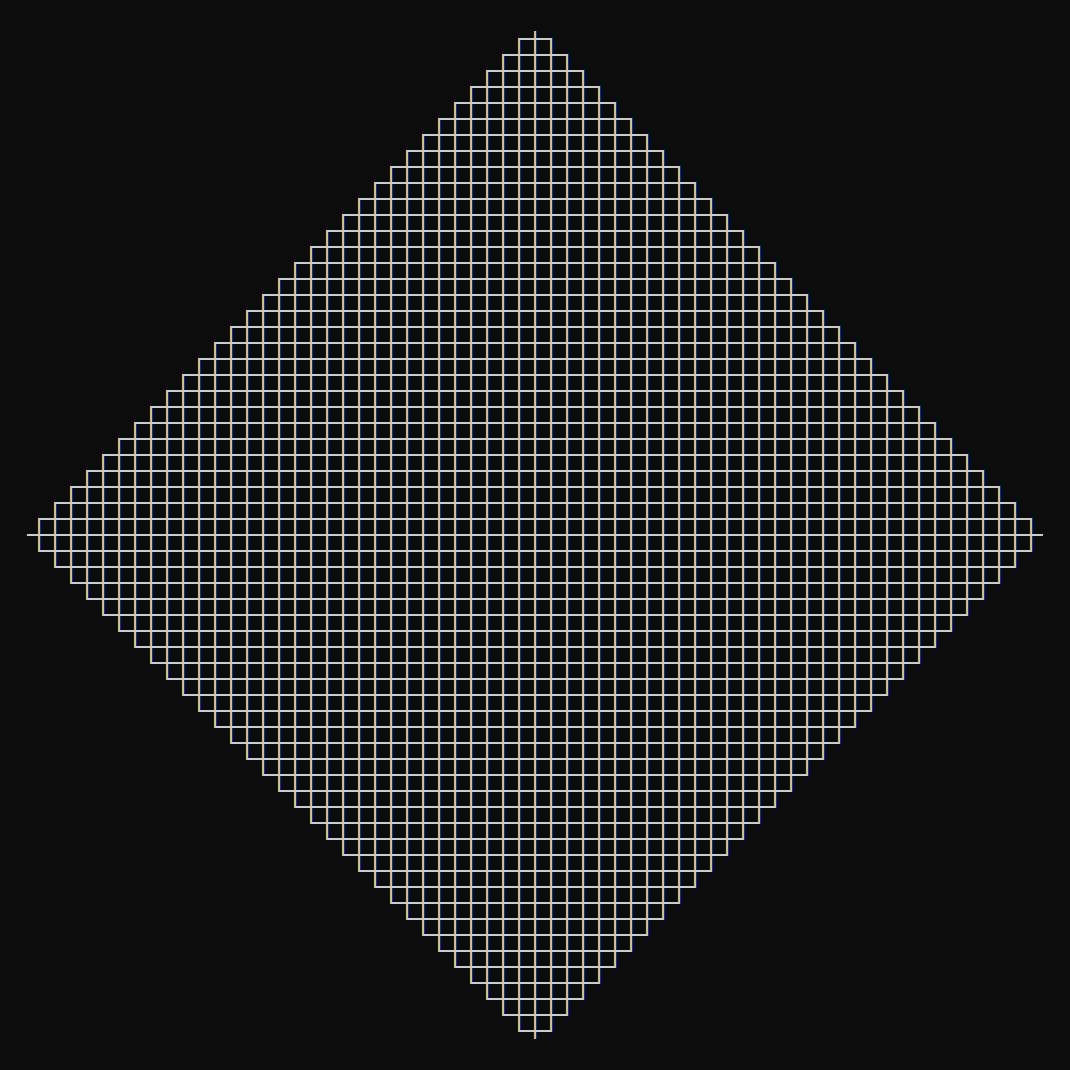Why the console? Because it's the cool way.

See All Fractals in the fractals-cli project.

## Usage

### Via `npx`:

``````\$ npx greek-cross-cli <n>
\$ npx greek-cross-cli <n> [size] [options]
``````

where `n >= 0` and `size >= n` (if provided).

### Via Global Install

``````\$ npm install --global greek-cross-cli
\$ greek-cross-cli <n>
\$ greek-cross-cli <n> [size] [options]
``````

where `n >= 0` and `size >= n` (if provided).

### Via Import

``````\$ npm install greek-cross-cli
``````

then:

``````const greek_cross = require('greek-cross-cli');
console.log(greek_cross.create(<n>);
console.log(greek_cross.create(<n>, {
size: <number>,
line: <bold|double|standard>
});
``````

The config params are optional.

## Options

### Recursive Step

``````\$ greek-cross-cli <n>
``````

The first param `<n>` is the recursive step. `<n>` should be an integer greater than or equal to 0.

#### Examples:

``````\$ greek-cross-cli 3
``````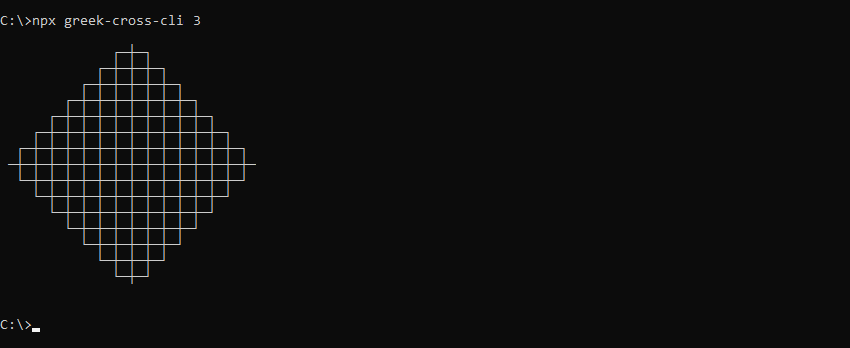``````\$ greek-cross-cli 4
``````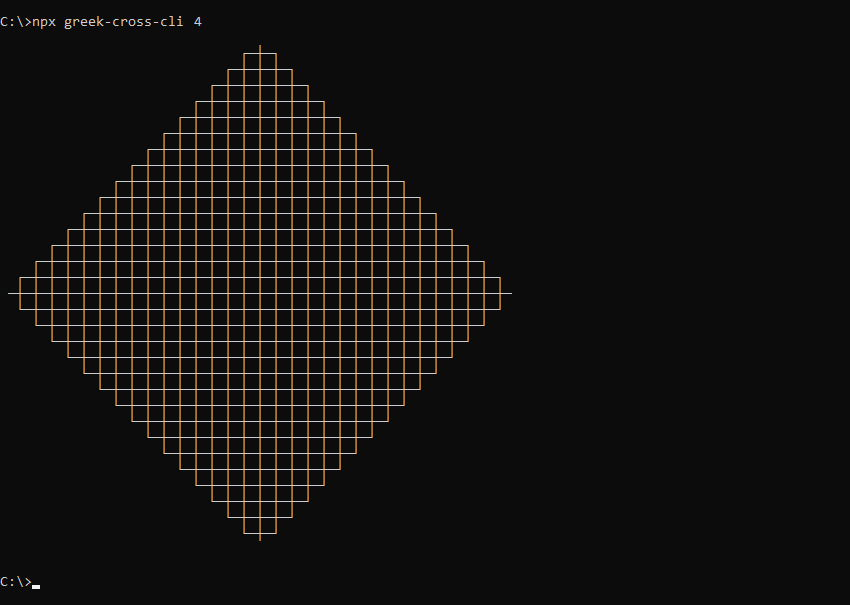### Size

``````\$ greek-cross-cli <n> [size]
``````

The optional `[size]` param allows the Greek Cross fractal to be drawn at larger sizes. `[size]` should be an integer greater than or equal to `<n>`. Including size will draw a Greek Cross fractal of `<n>` recursive steps the size of a Greek Cross fractal with `[size]` recursive steps.

#### Example:

``````\$ greek-cross-cli 2 4
``````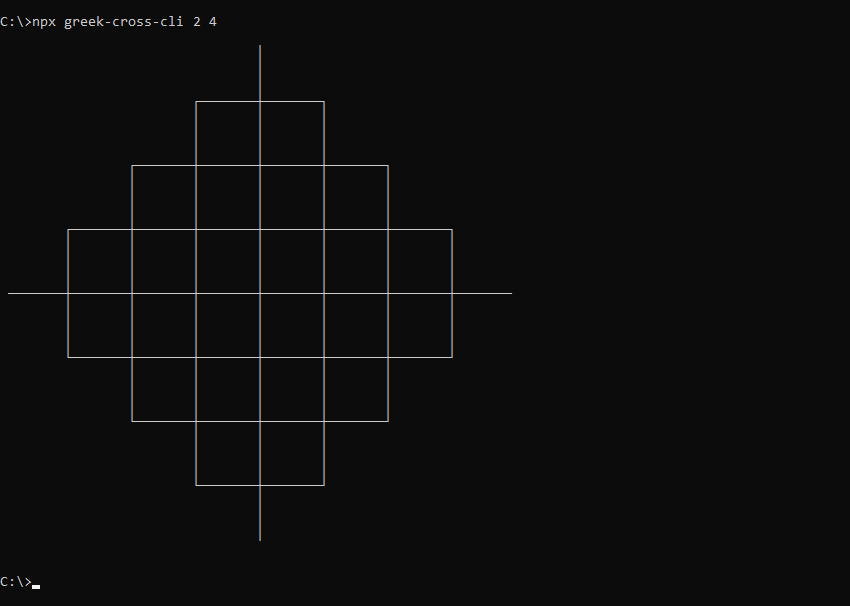### Line Type

``````\$ greek-cross-cli <n> --line=<bold|double|standard>
``````

The optional `--line` param draws the Greek Cross fractal using different line types. Supported values:

• `bold`: Draw using bold lines
• `double`: Draw using double lines
• `standard`: Draw using standard lines (default)

#### Examples:

``````\$ greek-cross-cli 4 --line=bold
``````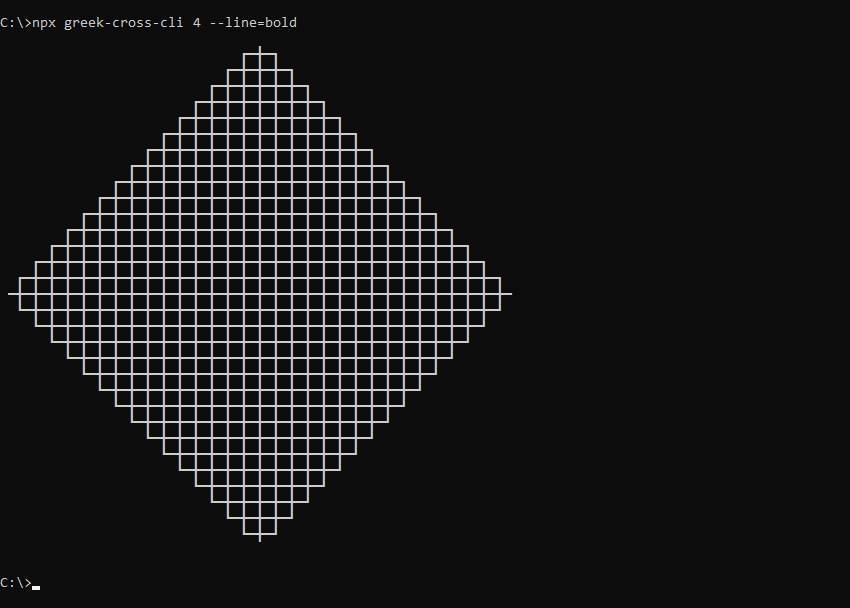``````\$ greek-cross-cli 4 --line=double
``````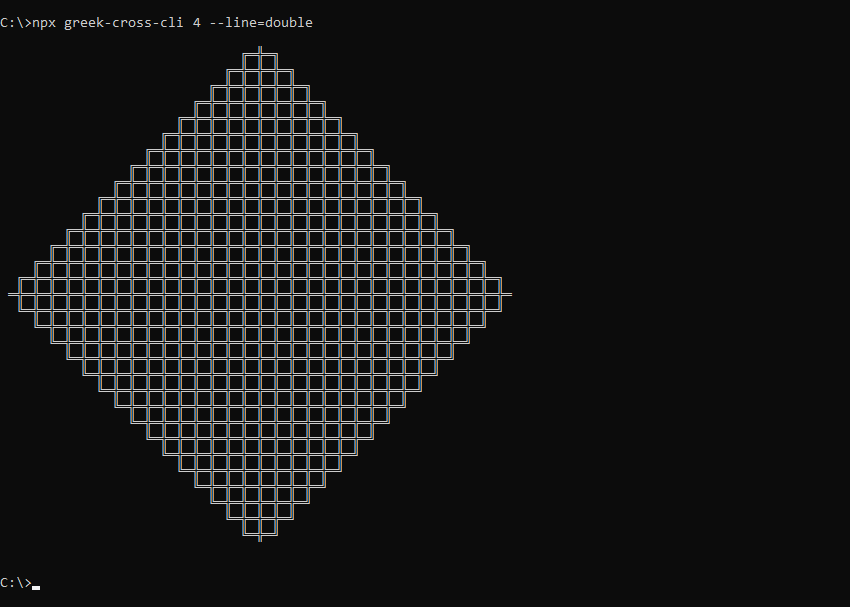## Package Sidebar

### Install

`npm i greek-cross-cli`

### Repository

github.com/spirometaxas/greek-cross-cli

### Homepage

spirometaxas.com/projects/fractals-cli

0

1.2.0

MIT

16.6 kB

5